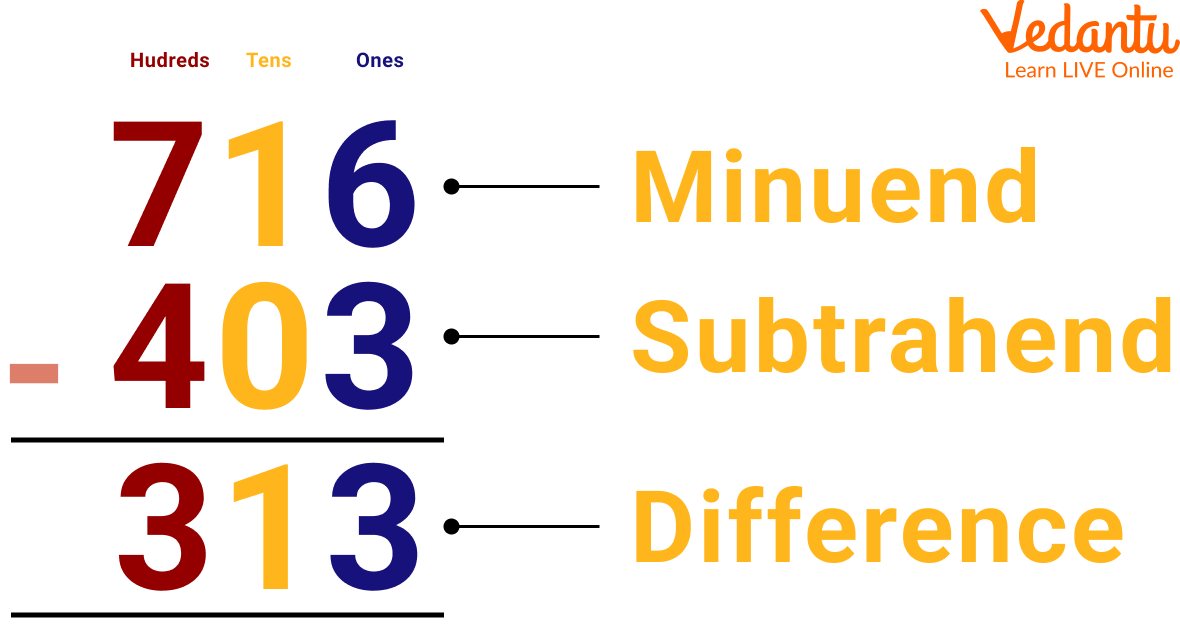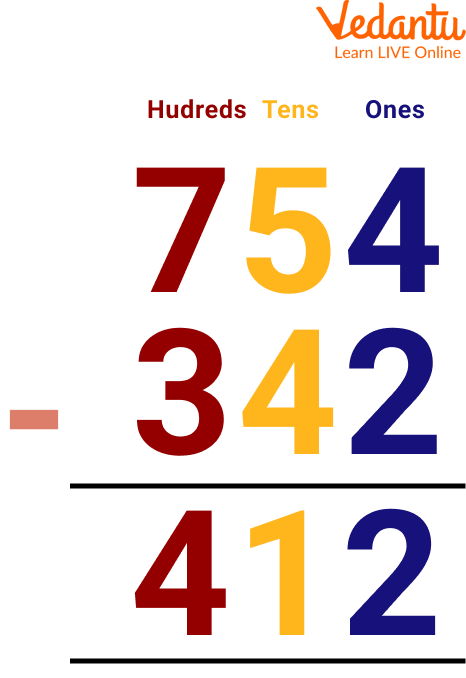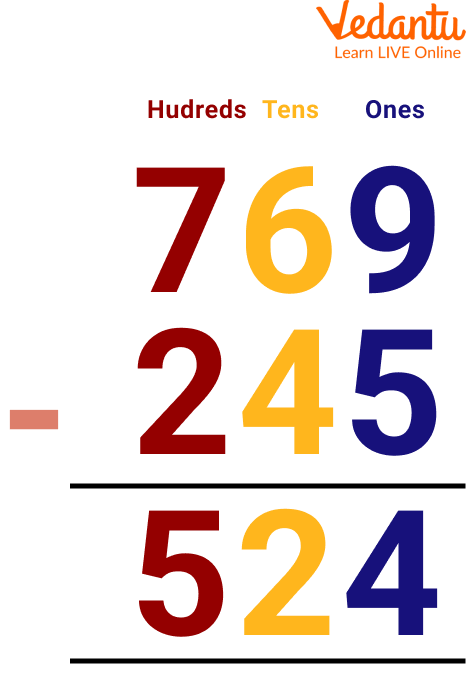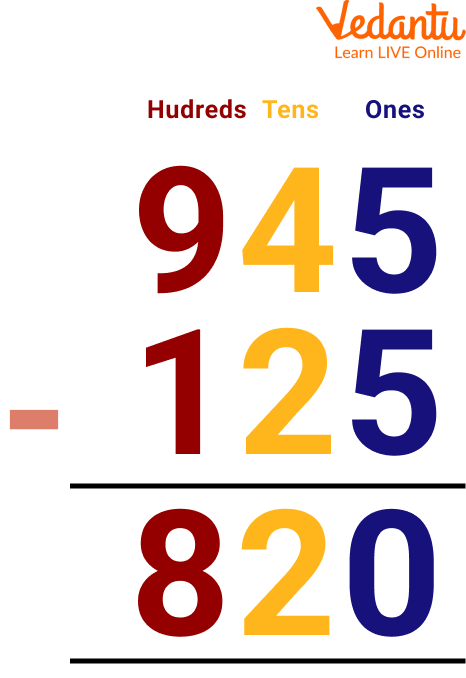Courses
Courses for Kids
Free study material
Free LIVE classes
More

# Subtraction of 3-Digit Numbers Without Regrouping## Introduction to 3-Digit Subtraction

3-digit subtraction is the subtraction of numbers in which the minuend consists of 3 digits and the subtrahend can be of either 1, 2, or 3 digits. We know that subtraction is an operation in Math, that gives the difference between two numbers. The number from which we subtract is called the minuend and the number which is subtracted from the minuend is called the subtrahend. While addition of numbers can be done with any set of numbers, in subtraction, we need to remember that the minuend as a whole should be larger than the subtrahend. In this article, we will discuss 3-digit subtraction without borrowing and the subtraction of 3-digit numbers without regrouping.

## What is 3-Digit Subtraction?

In 3-digit subtraction, we need to subtract the given numbers after placing them correctly according to their place values and we need to ensure that the bigger number is placed in the upper row, while the smaller number is placed below it. After aligning them into columns of ones, tens and hundreds, we start the process of subtraction. We know that the number from which the other number is subtracted is called the minuend, and the number which is subtracted from the minuend is called the subtrahend. The result of the subtraction of the two given numbers is called their difference.

Mathematically, this can be expressed as: Minuend - Subtrahend = Difference3-Digit Subtraction

## 3-Digit Subtraction Without Borrowing

3-digit subtraction without regrouping means subtracting a set of numbers without borrowing any number from the preceding digit. While subtracting 3-digit numbers, if all the digits in the minuend are bigger than the digits in the subtrahend, the subtraction can be easily done by subtracting each column one by one. Once all the columns are subtracted, the final answer is obtained. This is called subtraction without regrouping. In such a case, there is no regrouping or borrowing of numbers because all the digits of the minuend are bigger in value than the subtrahend.

For example, let us subtract two numbers to understand this better. Let us subtract 342 from 754.

Let us understand 3-digit subtraction without regrouping using the following steps:

Step 1: Subtract the numbers under the ones column. 4 - 2 = 2. Write the difference (2) in one column.

Step 2: Subtract the numbers under the tens column. 5 - 4 = 1. Write the difference (1) in the tens column.

Step 3: Subtract the numbers under the hundreds column. 7 - 3 = 4. Write the difference (4) in the hundreds column. Hence, the difference between the given numbers is 412.3-Digit Subtraction Without Borrowing

## Solved Examples on 3-Digit Subtraction Without Regrouping

Q1. Subtract the 3-digit numbers: 769 - 245.

Ans: Let us place the numbers one below the other and subtract them.

Step 1: Subtract the numbers under the ones column. 9 - 5 = 4. Write the difference (4) in one column.

Step 2: Subtract the numbers under the tens column. 6 - 4 = 2. Write the difference (2) in the tens column.

Step 3: Subtract the numbers under the hundreds column. 7 - 2 = 5 Write the difference (5) in the hundreds column.Subtract the 3-digit numbers: 769 - 245

This was three-digit subtraction without regrouping and the difference between the given numbers is 524.

Q2. Subtract the 3-digit numbers: 945 - 125.

Ans: Let us place the numbers one below the other and subtract them.

Step 1: Subtract the numbers under the ones column. 5 - 5 = 0. Write the difference (0) in one column.

Step 2: Subtract the numbers under the tens column. 4 - 2 = 2. Write the difference (2) in the tens column.

Step 3: Subtract the numbers under the hundreds column. 9 - 1 = 8. Write the difference (8) in the hundreds column.Subtract the 3-digit numbers: 945 - 125

This was three-digit subtraction without regrouping and the difference between the given numbers is 820.

## 3-Digit Subtraction Without Regrouping Practice Problems

Q1. Subtract the 3-digit numbers: 565 - 225.

Ans: 337

Q2. Subtract the 3-digit numbers: 845 - 123.

Ans: 722

Q3. Subtract the 3-digit numbers: 125 - 101.

Ans: 24

## Summary

In the above topic we have learnt the concept of subtraction and also how to solve 3-digit subtraction without borrowing and the subtraction of 3-digit numbers without regrouping. We have seen here to calculate the difference between the numbers using the number line. Also, we have solved some 3-digit subtraction without regrouping examples and 3-digit subtraction without regrouping solved problems for better clarity of the concepts. Hope this article will enhance your concept of subtraction of 3-digit numbers. To be more perfect in subtraction solve the above-given practice problems.

Last updated date: 27th Sep 2023
Total views: 78.6k
Views today: 2.78k

## FAQs on Subtraction of 3-Digit Numbers Without Regrouping

1. How do we subtract using regrouping?

Addition with regrouping is when one of the place value columns adds up to a number that is 10 or greater. Only the one digit is written down and the tens digit of the answer is regrouped to be added to the next column. Addition without regrouping is when the digits add up to a number that is 9 or less.

2. How can you relate subtraction and addition?

We need to know that subtraction is just the opposite of addition. When we add two numbers we get a greater number but if we calculate the difference between the two numbers then we get a lesser number.

3. Why is subtraction important in everyday life?

Subtraction is a part of our everyday lives and therefore an important concept to develop early. We need to understand how to subtract in order to engage with society effectively as we use subtraction when dealing with money, cooking, travel and time, among countless other daily experiences.